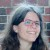# Find the unknown variable in the following. x/4=7/20​

Find the unknown variable in the following. x/4=7/20​

### 1 thought on “Find the unknown variable in the following. x/4=7/20​”

1.$$\frac{x}{4} = \frac{7}{20} \\ \\ 20x = 7 \times 4 \\ 20x = 28 \\ x = \frac{28}{20} \\ \\ x = \frac{7}{5} \: \:$$